# To match: The equation y = f ( x − 4 ) with the given graph and justify the choice of the graph.### Single Variable Calculus: Concepts...

4th Edition
James Stewart
Publisher: Cengage Learning
ISBN: 9781337687805### Single Variable Calculus: Concepts...

4th Edition
James Stewart
Publisher: Cengage Learning
ISBN: 9781337687805

#### Solutions

Chapter 1.3, Problem 3E

(a)

To determine

## To match: The equation y=f(x−4) with the given graph and justify the choice of the graph.

Expert Solution

The equation y=f(x4) is match with the graph 3.

### Explanation of Solution

The equation y=f(x4) represents that the graph of the function is shifted 4 units to the right side. Thus, the graph of y=f(x4) is shown below.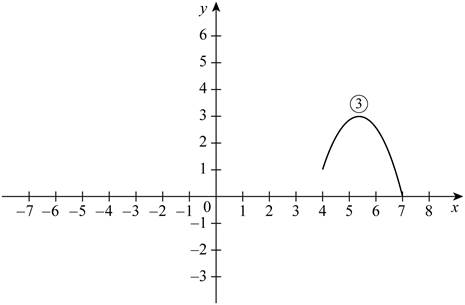Therefore, it can be concluded that the equation y=f(x4) matches with the graph 3.

(b)

To determine

### To match: The equation y=f(x)+3 with the given graph and justify the choice of the graph.

Expert Solution

The equation y=f(x)+3 is match with the graph 1.

### Explanation of Solution

The equation y=f(x)+3 represents that the graph of the function is shifted 3 units to the right side. Thus, the graph of y=f(x)+3 is shown below.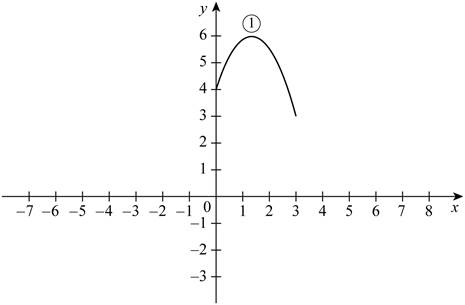Therefore, it can be concluded that the equation y=f(x)+3 matches with the graph 1.

(c)

To determine

### To match: The equation y=13f(x) with the given graph and justify the choice of the graph.

Expert Solution

The equation y=13f(x) is match with the graph 4.

### Explanation of Solution

The equation y=13f(x) represents that the graph of the function is shrunk vertically by a factor of 3. Thus, the graph of y=13f(x) is shown below.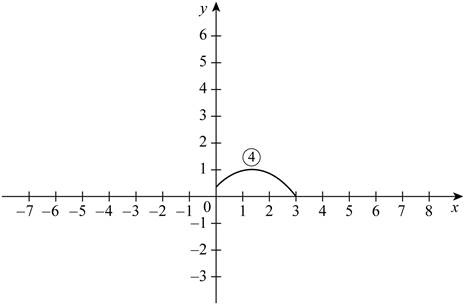Therefore, it can be concluded that the equation y=13f(x) matches with the graph 4.

(d)

To determine

### To match: The equation y=−f(x−4) with the given graph and justify the choice of the graph.

Expert Solution

The equation y=f(x4) is match with the graph 5.

### Explanation of Solution

The equation y=f(x4) represents that the graph of the function is shifted 4 units to the left side. Thus, the graph of y=f(x4) is shown below.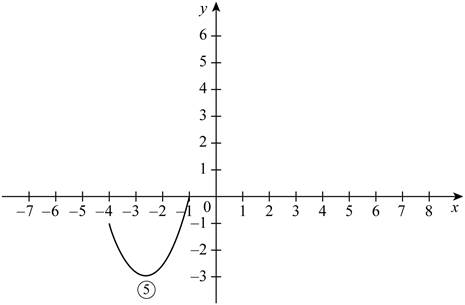Therefore, it can be concluded that the equation y=f(x4) matches with the graph 5.

(e)

To determine

### To match: The equation y=2f(x+6) with the given graph and justify the choice of the graph.

Expert Solution

Solution:

The equation y=2f(x+6) is match with the graph 2.

### Explanation of Solution

The equation y=f(x4) represents that the graph of the function is shifted 6 units to the left and stretched vertically by a factor of 2. Thus, the graph of y=f(x4) is shown below.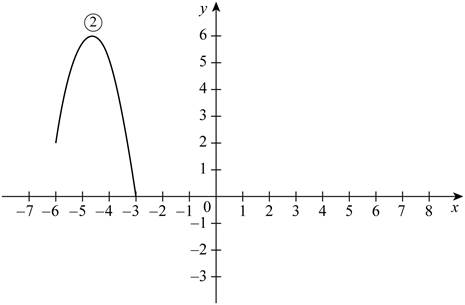Therefore, it can be concluded that the equation y=2f(x+6) matches with the graph 2.

### Have a homework question?

Subscribe to bartleby learn! Ask subject matter experts 30 homework questions each month. Plus, you’ll have access to millions of step-by-step textbook answers!